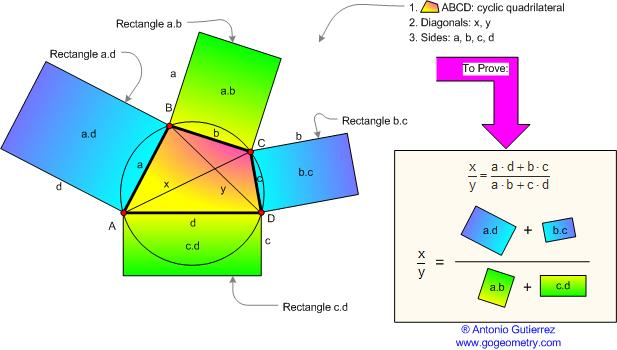Ptolemy's Theorem Extension. Level: High School, SAT Prep, College geometry In a cyclic quadrilateral the ratio of the diagonals equals the ratio of the sums of products of the sides that share the diagonals' end points. In other words, the ratio of the diagonals of any cyclic quadrilateral equals the ratio of the sums of the rectangles contained by the sides that share the diagonals' end points.Home | Geometry | Search | Problems | Quadrilateral | Ptolemy | Email | By Antonio Gutierrez